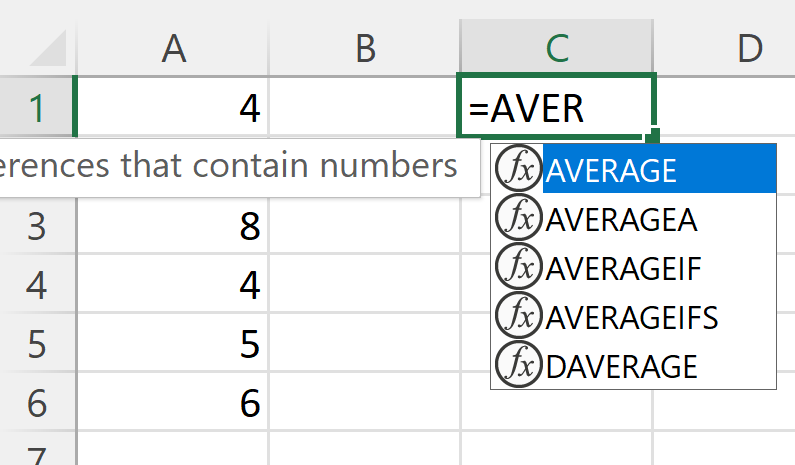# 3.7: Using Excel to Calculate Descriptive Statistics

$$\newcommand{\vecs}{\overset { \rightharpoonup} {\mathbf{#1}} }$$ $$\newcommand{\vecd}{\overset{-\!-\!\rightharpoonup}{\vphantom{a}\smash {#1}}}$$$$\newcommand{\id}{\mathrm{id}}$$ $$\newcommand{\Span}{\mathrm{span}}$$ $$\newcommand{\kernel}{\mathrm{null}\,}$$ $$\newcommand{\range}{\mathrm{range}\,}$$ $$\newcommand{\RealPart}{\mathrm{Re}}$$ $$\newcommand{\ImaginaryPart}{\mathrm{Im}}$$ $$\newcommand{\Argument}{\mathrm{Arg}}$$ $$\newcommand{\norm}{\| #1 \|}$$ $$\newcommand{\inner}{\langle #1, #2 \rangle}$$ $$\newcommand{\Span}{\mathrm{span}}$$ $$\newcommand{\id}{\mathrm{id}}$$ $$\newcommand{\Span}{\mathrm{span}}$$ $$\newcommand{\kernel}{\mathrm{null}\,}$$ $$\newcommand{\range}{\mathrm{range}\,}$$ $$\newcommand{\RealPart}{\mathrm{Re}}$$ $$\newcommand{\ImaginaryPart}{\mathrm{Im}}$$ $$\newcommand{\Argument}{\mathrm{Arg}}$$ $$\newcommand{\norm}{\| #1 \|}$$ $$\newcommand{\inner}{\langle #1, #2 \rangle}$$ $$\newcommand{\Span}{\mathrm{span}}$$$$\newcommand{\AA}{\unicode[.8,0]{x212B}}$$

Many future topics in this book require you to start by calculating some descriptive statistics, such as the mean and standard deviation, before completing the problem asked. To save time, this should be commonly completed using technology, in particular, using Excel.

This section will focus on using Excel formulas to do these calculations.

## Excel

Excel contains cells, each of which can contain some content. Each column is designated by a letter, and each row is designated by a number. In this way, we can refer to a specific cell by referring to the row and column that cell is in.Figure $$\PageIndex{1}$$: The number 5 is entered into cell A1. (Copyright; Katherine Bowe via Excel)

In the above figure, the number 5 is entered into cell A1.

When using Excel formulas, each element of the data can be used (for example, the 5 entered in the cell) or each cell name can be used (for example, the A1 where the 5 is entered in). For many instances, it saves time to use the cell name or reference instead of the actual data values.

## Mean

The Excel formula to calculate the mean is $$=\text{AVERAGE}()$$.

### Example $$\PageIndex{1}$$

Calculate the mean of the follow numbers using the Excel formula $$=\text{AVERAGE}()$$.

 4 6 8 4 5 6

Solution

Start by entering the data into Excel. This is generally done in a single column with each data value in a separate cell.Figure $$\PageIndex{2}$$: The data values entered into Excel in column A. (Copyright; Katherine Bowe via Excel)

Next, enter in the formula to use. As you start to type, Excel will provide suggestions to help you remember what formula to use.Figure $$\PageIndex{3}$$: Suggestion list in Excel when typing in the formula AVERAGE. (Copyright; Katherine Bowe via Excel)

Once you type in the open parenthesis, a reminder of the values Excel is expecting you to enter pops up.Figure $$\PageIndex{4}$$: Reminder of values to enter into formula. (Copyright; Katherine Bowe via Excel)

At this point, there are several options. The most basic way to finish entering the equation would be to enter all the data values:

$=\text{AVERAGE}(4,6,8,4,5,6)$

However, using this method requires you to enter every data value, and it is susceptible to incorrectly entering the values resulting in the incorrect result.

Cell references could also be used instead:

$=\text{AVERAGE}(A1,A2,A3,A4,A5,A6)$

This may help with reducing errors from typos, but it could be difficult to enter every cell reference. If the problem had asked to find the mean of 100 values rather than just 6, it would take some time to enter all that in.

The best method is to utilize the colon, which in Excel generally means "thru".

$=\text{AVERAGE}(A1:A6)$

Using this method reduces how many values need to be entered and reduces or eliminates all typos. Additionally, once you have typed the open parenthesis, $$=\text{AVERAGE}($$ you can actually just highlight all the data, then enter the close parenthesis, hit enter and Excel automatically enters the relevant cell references for you.

Once you have entered the formula and hit enter, the cell will contain the result of your calculation.Figure $$\PageIndex{5}$$: After using the formula, the cell contains the result of the calculation. (Copyright; Katherine Bowe via Excel)

In this example, the mean is 5.5. The cell C1, where the formula was entered only displays the result. But the formula entered can be seen above if you click on the cell. Using the formula to do the calculation is part of the problem, so when you turn in a spreadsheet, your instructor will click on the cells containing your results to see the formulas you used.

All the formulas discussed in this section use the same general method as discussed in the above example to complete the calculation.

## Measures of Center

The median and the mode can also be found using Excel formulas. The general method is the same as calculating the mean.

The formula for the median is

$=\text{MEDIAN}()$

The formula for the mode is

$=\text{MODE}()$

## Measures of Location

The minimum and the maximum can be found using Excel formulas. The general method is the same as calculating the mean.

The formula for the minimum is

$=\text{MIN}()$

The formula for the maximum is

$=\text{MAX}()$

There is not a formula to find range directly, however you can find the difference between the maximum and the minimum

$=\text{MAX}()-\text{MIN}()$

There are formulas that Excel uses for Quartiles and Percentiles, however, those formulas use a different method for calculation than what is presented in the earlier sections of this chapter, which gives different results. So we will not use Excel for calculating Quartiles or Percentiles.

The variance and the standard deviation can both be found using Excel formulas. The general method is the same as calculating the mean.

The first step for both of these is to decide if the data you have is a population or a sample. The calculation is different depending on this, so if you use the wrong formula, you will get the incorrect answer.

If your data is a population, then you can find the variance using the formula

$=\text{VAR.P}()$

and the standard deviation using the formula

$=\text{STDEV.P}()$

If your data is a sample, then you can find the variance using the formula

$=\text{VAR.S}()$

and the standard deviation using the formula

$=\text{STDEV.S}()$

3.7: Using Excel to Calculate Descriptive Statistics is shared under a CC BY license and was authored, remixed, and/or curated by LibreTexts.